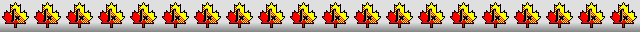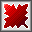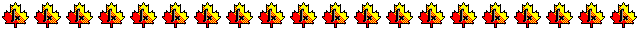Maple worksheets on the derivation of order 8 Runge-Kutta schemesOrder conditions for an order 8 Runge-Kutta scheme - RKcoeff8a.mws

• Order conditions for an order 8 Runge-Kutta scheme and their associated rooted trees.

Derivation of 11 stage, order 8 Runge-Kutta schemes - RKcoeff8b.mws

• Cooper-Verner schemes and Curtis schemes

See:
Some Explicit Runge-Kutta Methods of High Order, by G. J. Cooper and J. H. Verner,
SIAM Journal on Numerical Analysis, Vol. 9, No. 3, (September 1972), pages 389 to 405
and
An Eighth Order Runge-Kutta process with Eleven Function Evaluations per Step, by A. R. Curtis,
Numerische Mathematik, Vol. 16, No. 3  (1970), pages 268 to 277.

• Coefficients for the schemes and some properties
RKcoeff8b_1.pdf     RKcoeff8b_2.pdf     RKcoeff8b_3.pdf     RKcoeff8b_4.pdf

Derivation of 13 stage, combined order 7 and 8 Runge-Kutta schemes - RKcoeff8c.mws

Derivation of 13 stage, combined order 7 and 8 Runge-Kutta schemes - RKcoeff8d.mws

• The "classical" Prince and J.R.Dormand scheme together with similar schemes.
See: High order embedded Runge-Kutta formulae, by P.J.Prince and J.R.Dormand,
Journal of Computational and Applied Mathematics, vol. 7, 1981, pages 67-75.

• Coefficients schemes and some properties
RKcoeff8d_1.pdf     RKcoeff8d_2.pdf     RKcoeff8d_3.pdf

Procedures related to the construction of Runge-Kutta schemes - butcher.zip

Procedures for root-finding and determining minimum points  - roots.zip

Procedures for numerical integration  - intg.zip

Top of page

Main index